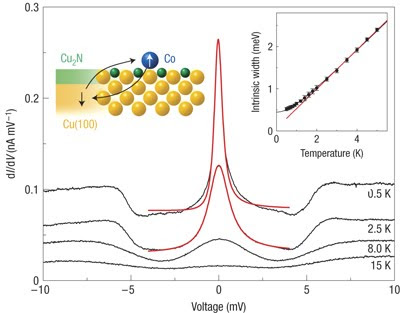Wednesday, April 14, 2010

Towards understanding the Kondo problem

This week's Quantum many-body theory reading group is reviewing some basics of scattering theory since that is so key to understanding some aspects of the Anderson single impurity model (Ch. 6 in Phillips) and the Kondo effect (Ch. 7).

Here are a few of the key ideas.
A nice treatment of some of the issues is also in Chapter 2 of The Kondo Problem to Heavy Fermions by A.C. Hewson.

Elastic scattering theory can be formulated in terms of phase shifts in different angular moment channels. The phase shift can be related to the T-matrix (equation 6.38).
Strongest scattering (unitary scattering) corresponds to a phase shift of pi/2.

When the scattering is strong one needs to calculate the T-matrix from the self-consistent equation
T = V + VGT (6.33)
where G is the full electron Greens function which is given by the Dyson equation
G=G0 + G0VG (6.32)
Both of these equations have nice diagrammatic interpretations.

John Fjaerestad, points out
there may be a typo in Eq. (6.33) in Phillips. I think G should replaced by G0 there. If one makes that change, one gets the same infinite series from Eq. (6.32) as from inserting (6.33) into (6.34). This definition of T is also consistent with other books I've checked, e.g. Eq. (7.2.18) in Sakurai where the Green's function involved contains H0, not H.
For the non-interacting Anderson model (or Hartree-Fock limit) unitary scattering occurs for electrons resonant with the impurity level.

The Friedel sum rule relates the occupation of the impurity level to the scattering phase shift for impurities at the Fermi energy (eqn. 6.42).

The Friedel sum rule reveals a major problem with the Hartree-Fock treatment of the Anderson model. Compare equations 7.71 and 7.72. The former (which is exact) implies that the impurity density of states at the Fermi energy should be much larger that that given by the latter. This was eventually resolved with the existence of the Kondo resonance peak at the Fermi energy. This is a highly non-trivial quantum many-body effect.This figure is taken from this paper.